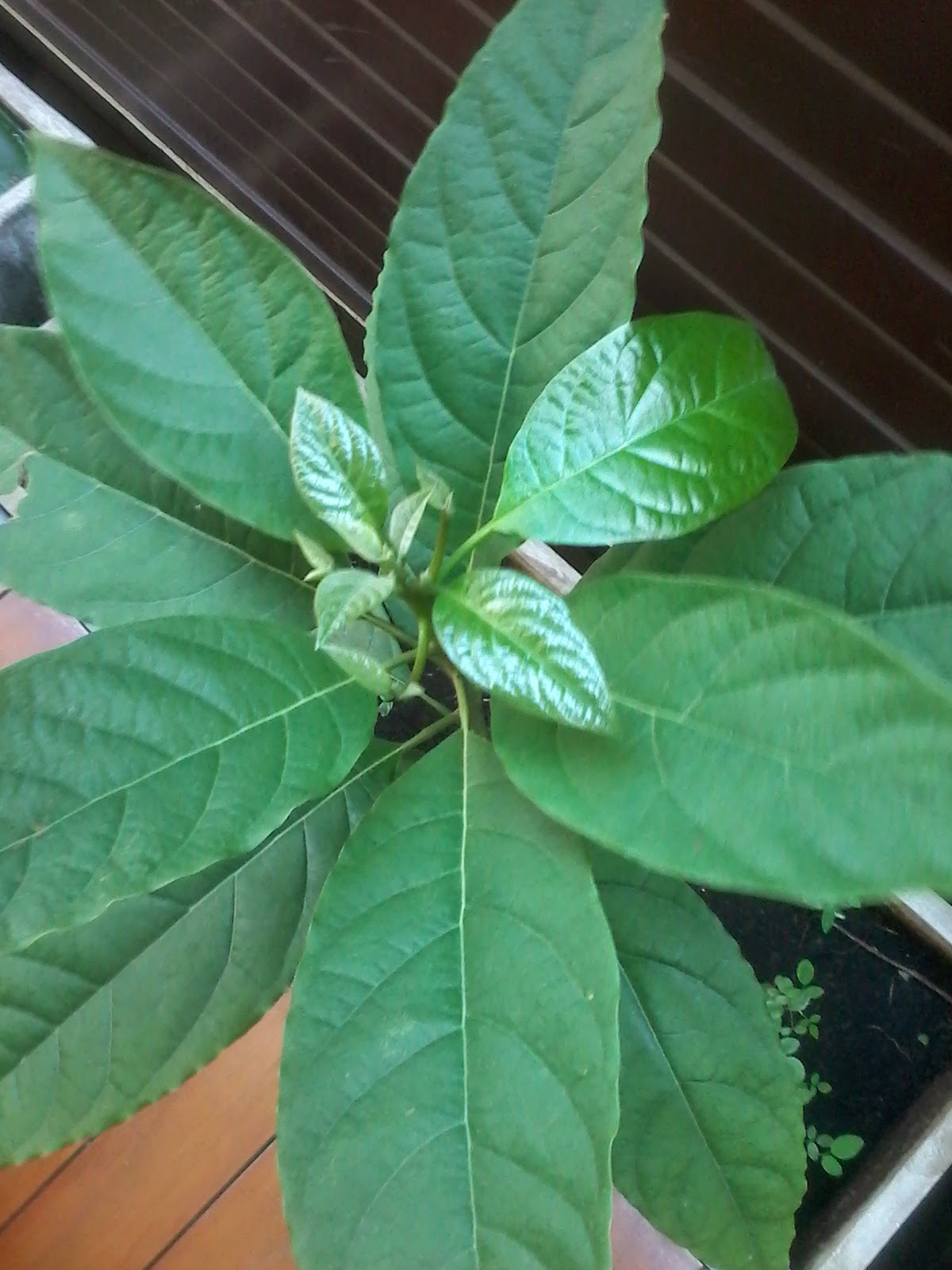## Tuesday, March 31, 2015

### Apologies to the avocado and sums of cubes

Who: J3 and J2
Where: side of the house
When: this afternoon

# Sums of Cubes

Cathy O'Neil (THE Mathbabe) flagged a proof-by-picture of Nichomachus's theorem yesterday and suggested it would be a fun discussion with kids. J2 and I started exploring it today. My current favourite introduction is to say: "a friend thought you might be interested to explore ..." In this case, to explore patterns from adding up perfect cubes.

From past conversations, his natural inclination was to start with 13, then 13+23, etc and look for patterns. Initially, he confused 23 and 24, so we clarified that and he embarked on a bunch of calculating. I kept notes for him. To give an easy extra term for his pattern seeking, I started with zero cubed.

First conjecture
We built our table to this level:
 03 = 0 03+13 = 1 03+13+23 = 9 03+13+23+33 = 36

At that point, J2 noticed we had squares and guessed that we were going to get every other square. Two nice conjectures, one of which already wasn't quite true, but that was more obviously clear with the next term:
 03 = 0 03+13 = 1 03+13+23 = 9 03+13+23+33 = 36 03+13+23+33+43 = 100 03+13+23+33+43+53 = 225

J0: so, are we still getting squares?
J2: ... yes. That's 15 squared
J0: hmm, shall we write that down?
J2: yes, daddy. write down 0 = 02, 1 = 12, 9 = 3 (etc)
J0: ok, so:
 03 = 0 = 02 03+13 = 1 = 12 03+13+23 = 9 = 32 03+13+23+33 = 36 = 62 03+13+23+33+43 = 100 = 102 03+13+23+33+43+53 = 225 = 152
J2: hey, those are triangular numbers!

More testing
For the rest of the conversation, he talked about what he expected the next terms would be, then he did the calculations to check. He didn't remember 6 cubed or 7 cubed, so we had diversions to talk about strategies to calculate them. At the end, he was very excited to see that the conjecture was still working, our cubes were adding up to squares of triangular numbers.

To be continued
Frankly, I think it will be a while before he can attack the wallet proof on his own (or with my minimal guidance). In the next couple of weeks, if we have access to some blocks construction sets, though, I'm hoping we can work together to actually do these transformations, rearranging the cubes into squared triangular numbers and vice versa. I expect even this will be a bit difficult, but it should be fun!How tall is it? Shoulder heightKey observation: these new leaves are very shiny
1.